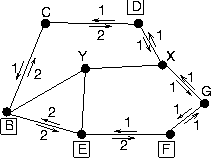### 1 [5 pts]. Solution

Generator is 10011. So r=4.

```                 11111100
______________
10011 | 111011000100
10011
-----
11101
10011
-----
11100
10011
-----
11110
10011
-----
11010
10011
-----
10011
10011
-----
00000
00000
-----
00000
00000
-----
0000

It is acceptable because the remainder is 0000.
The data bit sequence is 11101100 (111011000100 minus last 4 bits).
```

• 4 points for the division, 1 point for the data sequence.

### 2 [10 pts]. Solution

```Time    At B, distance to D      At C, distance to D
via node C              via node B  via node D

0.1           4*                      6        2*
1.0           4*                      6*      11

1.1           8*                      6*      11

2.1           8*                     10*      11

3.1          12*                     10*      11

4.1          12*                     14       11*

5.1          13*                     14       11*

6.1          13*                     15       11*

* indicates the minimum distance entry

Stable at time 6.1.
```

• Entry at time 1.0 did not count toward grade.
• Max 2 points for stating that stable by time 2.1 or 3.1.
• Max 6 points for not getting it correct but showing that the min distance decreases by 4 every iteration.
• Lose 1 point if everything correct but stabilization time.
• Lose 2 points if only one line is wrong.

### 3 [10 pts]. Solution

a.b.c.• Part a: 2 points for spanning tree. 2 points for aggregate flows.
• Part b: 1 point for each spanning tree.
• Part c: 2 points for aggregate flows.

### 4 [5 pts]. Solution

Below, * denotes multiplication and ** denotes exponentiation.

For slotted-ALOHA of N nodes, the throughput (as fraction of successful slots) is N*p*[(1-p)**(N-1)], where p is transmission probability. Thus maximum throughput is (1-p)**(N-1), which is achieved when p=1/N.

The 4-user group and even slots form a 4-node slotted-ALOHA system. Thus its max throughput is achieved when p=(1/4), and equals (1 - (1/4))**3, which equals (3/4)**3, which equals 27/64.

The 26-user group and odd slots form a 26-node slotted-ALOHA system. Thus its max throughput is achieved when p=(1/26), and equals (1 - (1/26))**25, which practially equals (1/e).

Thus the overall throughput is (1/2)[(27/64) + (1/e)]